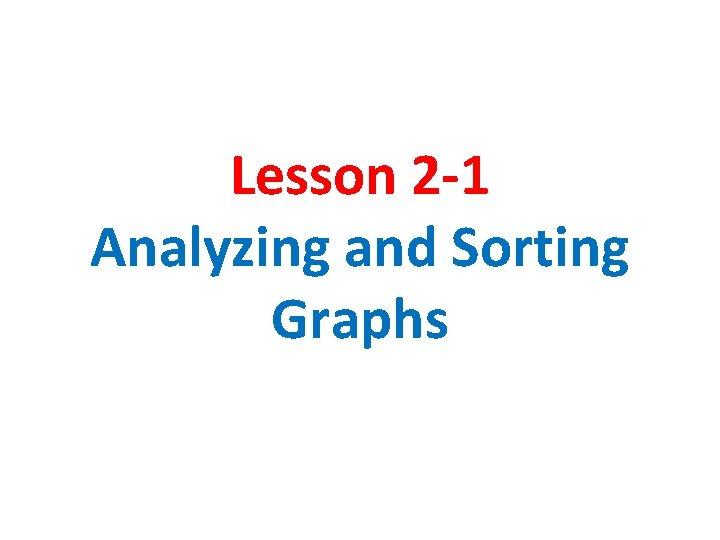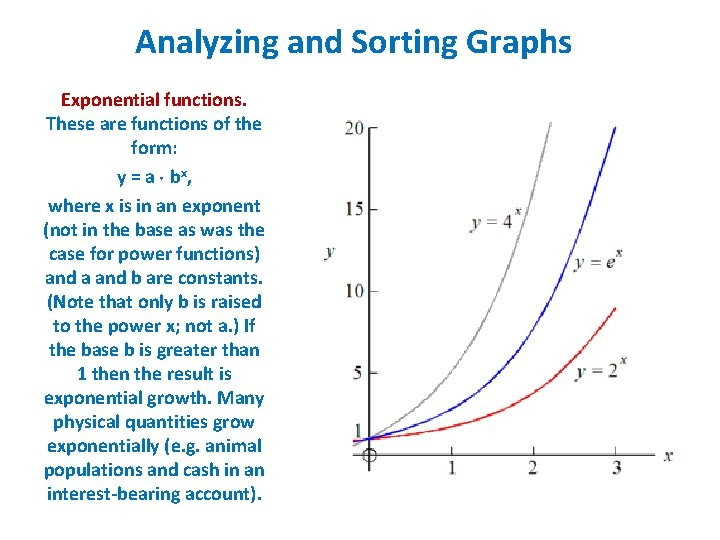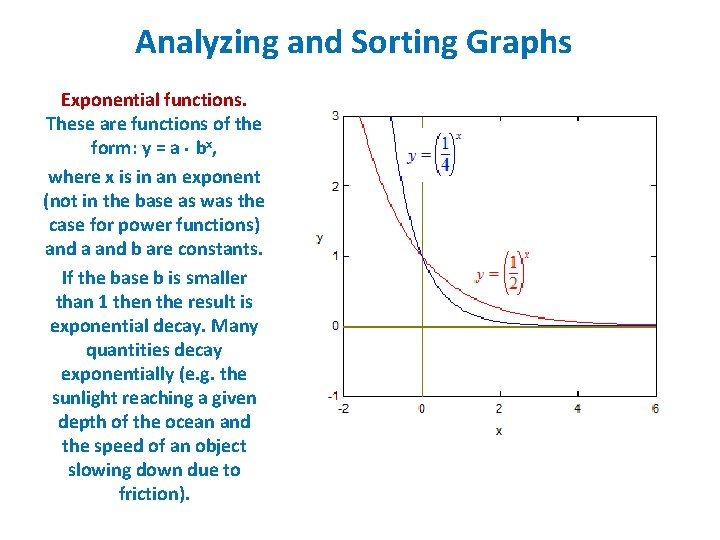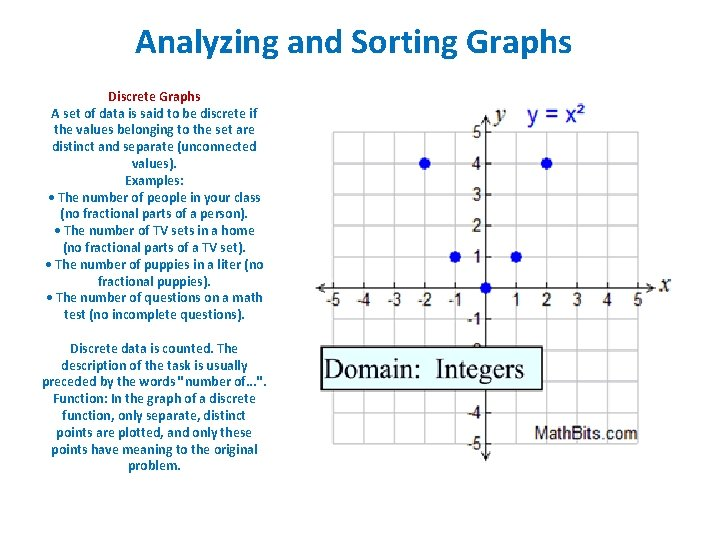Lesson 2 1 Analyzing and Sorting Graphs Analyzing

• Slides: 7Lesson 2 -1 Analyzing and Sorting GraphsAnalyzing and Sorting Graphs Linear functions These are functions of the form: y = mx + b, where m and b are constants. A typical use for linear functions is converting from one quantity or set of units to another. Graphs of these functions are straight lines. m is the slope and b is the y intercept. If m is positive then the line rises to the right and if m is negative then the line falls to the right.Analyzing and Sorting Graphs Quadratic functions These are functions of the form: y = ax 2 + bx + c, where a, b and c are constants. Their graphs are called parabolas. This is the next simplest type of function after the linear function. Falling objects move along parabolic paths. If a is a positive number then the parabola opens upward and if a is a negative number then the parabola opens downward.Analyzing and Sorting Graphs Exponential functions. These are functions of the form: y = a bx , where x is in an exponent (not in the base as was the case for power functions) and a and b are constants. (Note that only b is raised to the power x; not a. ) If the base b is greater than 1 then the result is exponential growth. Many physical quantities grow exponentially (e. g. animal populations and cash in an interest-bearing account).Analyzing and Sorting Graphs Exponential functions. These are functions of the form: y = a bx, where x is in an exponent (not in the base as was the case for power functions) and a and b are constants. If the base b is smaller than 1 then the result is exponential decay. Many quantities decay exponentially (e. g. the sunlight reaching a given depth of the ocean and the speed of an object slowing down due to friction).Analyzing and Sorting Graphs Continuous Graph Definition: A set of data is said to be continuous if the values belonging to the set can take on ANY value within a finite or infinite interval. Examples: • The height of a horse (could be any value within the range of horse heights). • Time to complete a task (which could be measured to fractions of seconds). • The outdoor temperature at noon (any value within possible temperatures ranges. ) • The speed of a car on Route 3 (assuming legal speed limits). Continuous data usually requires a measuring device. (Ruler, stop watch, thermometer, speedometer, etc. ) Function: In the graph of a continuous function, the points are connected with a continuous line, since every point has meaning to the original problem.Analyzing and Sorting Graphs Discrete Graphs A set of data is said to be discrete if the values belonging to the set are distinct and separate (unconnected values). Examples: • The number of people in your class (no fractional parts of a person). • The number of TV sets in a home (no fractional parts of a TV set). • The number of puppies in a liter (no fractional puppies). • The number of questions on a math test (no incomplete questions). Discrete data is counted. The description of the task is usually preceded by the words "number of. . . ". Function: In the graph of a discrete function, only separate, distinct points are plotted, and only these points have meaning to the original problem.